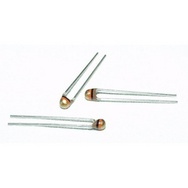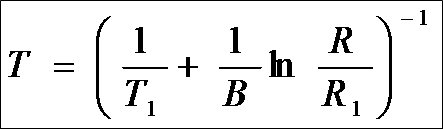# Measure temperature

There are many ways to measure temperature with an Arduino. We will discuss how to use a thermistor and a temperature IC.

Here is a picture of a 10K thermistor:Here is a picture of a temperature IC, LM35:The principle behind a thermistor (or the sensing component of a temperature IC) is the physics of semiconductors. A thermistor is a heavily-doped semiconductor. A semiconductor is conducting weakly compared with a conductor like copper but much more conductive compared with insulators like rubber.

The ability to conduct depends on a few factors. Among them, the amount of conducting charges in the material is quite important, which is directly proportional to the conductivity of the material. Insulators have very little conducting charges but conductors have a lot. The amount of charges in these two types of material don’t change much near room temperature. Semiconductors on the other hand, have moderate amount of conducting charges, whose quantity changes very largely as temperature changes. At high temperature, a semiconductor releases more conduction charges, it is less resistive. At low temperature, it releases less conduction charges and is thus more resistive. If you dope a semiconductor, the change of resistance becomes more pronounced for easy measurement.

So to sum it up, a thermistor is a resistor, whose resistance value decreases with increasing temperature, the therm- and -istor. This is like transistor is trans- and -istor. To measure temperature, we measure the resistance of a thermistor with a voltage divider. Let’s build a voltage divider and measure some temperatures.

First, purchase some 10KOhm thermistors for any electronics vendor. They should provide you the temperature vs. resistance data or formula to calculate temperature from resistance.

Then build a voltage divider and put a 10KOhm resistor in series with the thermistor. Connect 5V to the resistor and GND to the thermistor.Now the voltage at the junction between the resistor and thermistor will change when ther thermistor changes resistance at a change of temperature. The more resistive, the higher the voltage.

Connect an analog channel to the junction. You can then sense the voltage and output to PC or display on an LCD screen.

Test the above parts with a multimeter before attempting to program Arduino to display temperature.

To display temperature, you may copy down the resistance of your 10KOhm thermistor at all different temperatures into an array.

Then read the voltage, convert voltage into resistance from simple circuit calculations.

R=V/(5-V)*10KOhm

If you care about error bar, read my Resistor sorter theoretical error.

Every time you read in a voltage, convert it into resistance, then look up the table for two values that are closest to your reading, use interpolation to find a more exact temperature value. You can also use the formula provided to you by the manufacturer to estimate the temperature like this one:

or a simplified version:Formula 2 (Simplified formula that gives resistance at temperature T(k))

You need to invert the function obviously to get temperature:Formula 3 (Simplified formula that gives temperature T(k) at certain resistance R(ohm))

The values of T1, R1, and B should be given to you in the spec sheet, such as T1=298K (ie. 25DegC), R1=10K (the resistance of the thermistor at T1 temperature, so a “10K” thermistor will have R1=10K at 25DegC), B=4050K. The calculated result is in Kelvin. After subtracting 273 you will get DegC.

If this is too much math for you, I have good news, the temperature IC makes it easier to read temperature:

You may also use a temperature IC. Most common temperature ICs are either calibrated for degree C or F, like the LM35 is calibrated to degree C. They output a voltage proportional to these temperatures, both positive and negative, say 10mV/C or 10mV/F. Connect the output of the IC to Arduino analog input and you are good to go. Arduino can’t measure negative voltages so you either have to limit your measurement above 0DegC or 0DegF, or you can find one that reports Kelvin, which never reaches zero. Another way to measure negative voltage is to shift it to positive voltage with a bipolar to unipolar converter circuit. You will need a few things to construct a converter, which I can cover in a different post.

Notes:

If you are measuring temperature near where your arduino is, the LM35 gives the easiest way to achieve that. On the other hand, if you measure temperature at a distance and have to run a long wire between arduino and the temperature sensor, you should use a thermistor to reduce noise. You can always pick the best serial resistor for your thermistor so the output voltage is neither too high nor too low.

More on discrete thermistors:

You need to choose the right fixed resistor for optimal accuracy. First determine your range of temperature you measure first, then find the middle of that range, and pick a fixed resistor that has the same resistance as the thermistor if it were at the middle of the temperature range. IE. if your temperature measurement is around 25DegC and you are using a 10Kohm thermistor, you need a 10Kohm fixed resistor in series to the thermistor. If you use a say 200Kohm thermistor with R1=200Kohm and B=4022K (use formula 2 and 3), and your temperature range is -30DegC to 50DegC, so center is 10DegC, then you need a fixed resistor that matches the thermistor resistance at 10DegC, which is 409Kohm according to formula 2. So you either use 390Kohm or 430Kohm as both are standard values easy to obtain.

There exist applications of heating up thermistors with large current. If you want to tell liquid level, you can use the liquid as heat sink and apply larger current on the thermistors so only those ones in the liquid will stay cool and have large resistance. The ones outside liquid are hot and less resistive.

Some simple code for 10Kohm thermistor sensing:

void setup()

{

Serial.begin(9600);

}

void loop()

{

float resistance, voltage, r_fixed, T0, T, R0,B;
T0 = 298.15;//initial temperature for calibration
R0 = 10000;//initial resistance for calibration
r_fixed = 10972;//known resistance
B = 4050;//B for normalization
Serial.println(“Temperature”);

while(1)
{
voltage = analogRead(sensor_a)*5.0/1024;// Renormalizing from 0 to 5 volts.
resistance = voltage*r_fixed/(5.00-voltage);//find unknown resistance
T = 1/(log(resistance/r_fixed)/B+1/T0)-273.14;//find temperature from unknown ressistance
delay(500);
Serial.println(T);

}
}

### 3 Responses to Measure temperature

1.toilet tank says:

Hey there! I know this is kinda off topic but I was wondering if you knew where
I could locate a captcha plugin for my comment form?
I’m using the same blog platform as yours and I’m having difficulty finding one?
Thanks a lot!

•liudr says:

Not sure if this is spam. I don’t have captcha on comments. This is a free wordpress blog. Whatever comes with it is what I am using.